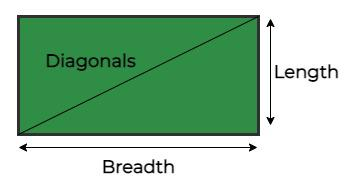GFG App
Open AppBrowser
Continue

# C++ Program to Find Diagonal of a Rectangle

Given two positive integers i.e, the length and breadth of the rectangle, the task is to find the length of the Diagonal of a Rectangle.Example:

output: The Diagonal is 5

The diagonal of a rectangle is sqrt (a*a+ b*b)

## C++

 `// C++ Program to Find Diagonal of a Rectangle ` `#include ` ` `  `using` `namespace` `std; ` ` `  `// Function to find the length of the ` `// diagonal of a rectangle of given sides ` `double` `findDiagonal(``double` `a, ``double` `b) ` `{ ` `    ``return` `sqrt``(a * a + b * b); ` `} ` ` `  `// Driver Code ` `int` `main() ` `{ ` `    ``double` `a = 4, b = 3; ``// a is the length and b is the ` `                         ``// breadth of the rectangle ` `    ``cout << ``"The Diagonal is "` `<< findDiagonal(a, b); ` ` `  `    ``return` `0; ` `}`

Output

`The Diagonal is 5`

Time Complexity: O(1)

Auxiliary Space: O(1)

My Personal Notes arrow_drop_up